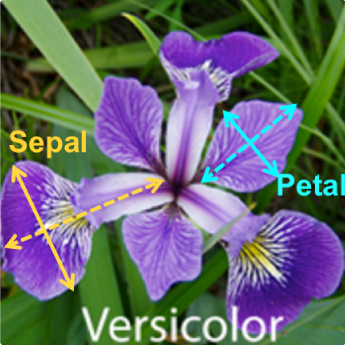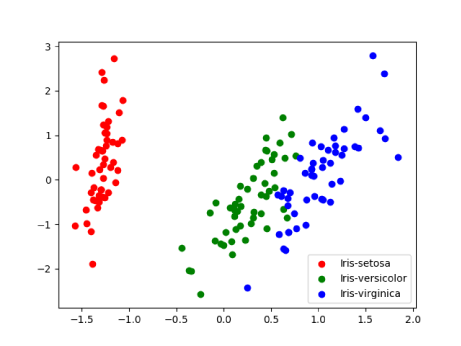# A Sample Higher Dimensional Dataset

### Petals are modified leaves that surround the reproductive parts of flowers. They are often brightly colored or unusually shaped to attract pollinators. Where Sepals are a little support structure underneath the petal which typically function as protection for the flower in bud. Below diagram shall give fair idea about petals, sepals and their length-width.### Based on the combination of these four features, Iris flower is classified into different 3 species. For example,

Flower# Sepal Length Sepal Width Petal Length Petal Width Species
1 5.1 3.5 1.4 0.2 Iris setosa
2 4.9 3.0 1.4 0.2 Iris setosa
3 7.0 3.2 4.7 1.4 Iris versicolor
4 6.4 3.2 4.5 1.5 Iris versicolor
5 6.7 3.3 5.7 2.5 Iris virginica
6 6.7 3.0 5.2 2.3 Iris virginica

# Pre-requisites for hands-on

### Python packages to be deployed are:

Package Name Usage
scikitlearn Opensource having simple and efficient tools for data mining and
data analysis. It leverages below numpy, scipy internally.
numpy Fundamental package for scientific computing using python.
Sophisticates managing N-dimensional array object.
scipy open-source software for mathematics, science, and engineering
matplotlib For comprehensive 2D plotting and data visualizing
pandas  Open source, BSD-licensed library providing high-performance, easy-to-use data structures and data analysis tools for the Python programming language. Helps reading data from different ﬁle formats into in-memory dataframes.

# SVM (Support Vector Machine) – For Multivariate Dataset Classification

### Note: You can run the program from command line using “python svm_for_multivariate_data.py”

“””
This code demonstrates SVM(Support Vector Machine) for classification of multi-dimensional Dataset.
Please refer here a sample dataset of Iris flowers having multiple dimensions i.e.
petal-length, petal-width, sepal-length, sepal-width.

You can do “wget https://archive.ics.uci.edu/ml/machine-learning-databases/iris/iris.data” to save this dataset locally. Or optionally you can also refer this URL directly while loading dataframe.
“””

import pandas as pd
from sklearn import model_selection
from sklearn import svm

# Read dataset into pandas dataframe
df = pd.read_csv(‘/your_path_for_input_dataset/iris.data’,names=[‘sepal-len’, ‘sepal-width’, ‘petal-len’, ‘petal-width’,’target’])
iris_features = [‘sepal-len’, ‘sepal-width’, ‘petal-len’, ‘petal-width’]

# Extract features
X = df.loc[:, iris_features].values

# Extract target i.e. iris species
Y = df.loc[:, [‘target’]].values

# Now using scikit-learn model_selection module, split the iris data into train/test data sets

# keeping 40% reserved for testing purpose and 60% data will be used to train and form model.
X_train, X_test, Y_train, Y_test = model_selection.train_test_split (X, Y, test_size=0.4, random_state=0)

# Build an SVC (Support Vector Classification) model using linear regression
clf_ob = svm.SVC(kernel=’linear’, C=1).fit(X_train, Y_train)

### But how do we know our model built is robust or accurate enough. We can measure this model performance using the reserved test data.

print(clf_ob.score(X_test, Y_test))

# So, how to validate the model robustness? Use K-Fold Cross Validation technique

### Here is code snippet for K-Fold cross validation against the SVC model with linear kernel constructed for the Iris dataset.

# Further, let’s now validate robustness of above model using K-Fold Cross validation technique

# We give cross_val_score a model, the entire iris data set and its real values, and the number of folds:

scores_res = model_selection.cross_val_score(clf_ob, X, Y, cv=5)

# Print the accuracy of each fold (i.e. 5 as above we asked cv 5)
print(scores_res)

# And the mean accuracy of all 5 folds.
print(scores_res.mean())

### Here cv=5 stands for use 5 folds or runs using different training datasets. This code snippet gives 5 folds score something like below and mean score around 0.98 which denotes the model is indeed working pretty good. Notice that it is greater than score 0.966666666667 discovered earlier using one pass.

[0.96666667, 1., 0.96666667, 0.96666667, 1.]

0.98

# Predict category of new data

### This model now can be used to predict new Iris flowers species using their given sepal length-width and petal length-width. Let’s use below dataset. Their species are given for reference. The model shall predict the species correctly.

Flower# Sepal Length Sepal Width Petal Length Petal Width Species
1 4.9876 3.348 1.8488 0.2 Iris setosa
2 5.3654 2.0853 3.4675 1.1222 Iris versicolor
3 5.890 3.33 5.134 1.6 Iris virginica

### Append below python code lines and run the program.

in_data_for_prediction = [[4.9876, 3.348, 1.8488, 0.2], [5.3654, 2.0853, 3.4675, 1.1222], [5.890, 3.33, 5.134, 1.6]]

p_res = clf_ob.predict(in_data_for_prediction)
print(‘Given first iris is of type:’, p_res)
print(‘Given second iris is of type:’, p_res)
print(‘Given third iris is of type:’, p_res)

### Indeed, it gives expected output:

Given first iris is of type: Iris-setosa
Given second iris is of type: Iris-versicolor
Given third iris is of type: Iris-virginica

# Dimensionality Reduction concept and associated technique

### Below code snippet transforms 4-D into 2-D dataset; prints preserved variance of original dataset which comes around 0.977631775025 which is quite good (x->1 is good).

“””
Dimensionality Reduction using PCA (Principal Component Analysis) Here n_components = 2 means, transform into a 2-Dimensional dataset.
“””
pca = PCA(n_components=2, whiten=True).fit(X)
X_pca = pca.transform(X)
print(‘explained variance ratio:’, pca.explained_variance_ratio_)
print(‘Preserved Variance:’, sum(pca.explained_variance_ratio_))

# Print scatter plot to view classification of the simplified dataset
colors = cycle(‘rgb’)
target_names = [‘Iris-setosa’, ‘Iris-versicolor’, ‘Iris-virginica’]

pl.figure()

target_list = array(Y).flatten()
for t_name, c in zip(target_names, colors):

pl.scatter(X_pca[target_list == t_name, 0], X_pca[target_list ==t_name, 1], c=c, label=t_name)

pl.legend()
pl.show()

### Original Dataset Records:

Flower# Sepal Length Sepal Width Petal Length Petal Width Species
0 5.1 3.5 1.4 0.2 Iris setosa
70 5.9 3.2 4.8 1.8 Iris versicolor
120 6.9 3.2 5.7 2.3 Iris virginica

### Transformed Dataset Records:

Flower# X axis Y axis Species
0 -1.3059028 0.66358991 Iris-setosa
70 0.5430661 -0.17110324 Iris-versicolor
120 1.18133597 0.76553312 Iris-virginica

### Using matplotlib library, a scatter plot is printed for this transformed data which looks something like below. Run the program to view preserved variance and scatter plot.